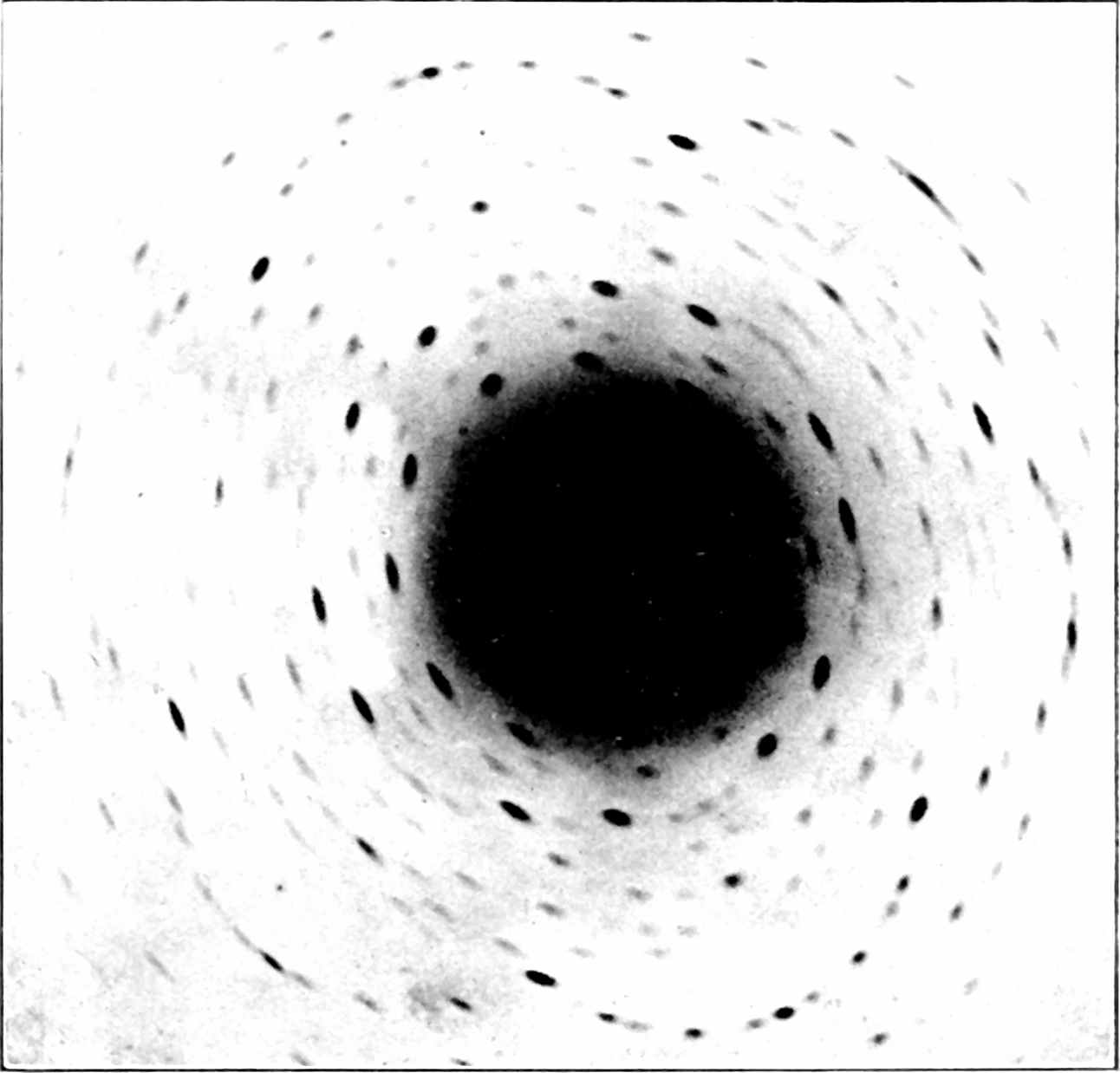# Wiener theorem

## Now with bonus Bochner!The special deterministic case of the Wiener-Khintchine theorem, written up with a slightly different notation for a slightly different project.

$\renewcommand{\var}{\operatorname{Var}} \renewcommand{\dd}{\mathrm{d}} \renewcommand{\pd}{\partial} \renewcommand{\bb}{\mathbb{#1}} \renewcommand{\vv}{\boldsymbol{#1}} \renewcommand{\mm}{\mathrm{#1}} \renewcommand{\mmm}{\mathrm{#1}} \renewcommand{\cc}{\mathcal{#1}} \renewcommand{\ff}{\mathfrak{#1}} \renewcommand{\oo}{\operatorname{#1}} \renewcommand{\gvn}{\mid} \renewcommand{\II}{\mathbb{I}\{#1\}} \renewcommand{\inner}{\langle #1,#2\rangle} \renewcommand{\Inner}{\left\langle #1,#2\right\rangle} \renewcommand{\finner}{\langle #1,#2;#3\rangle} \renewcommand{\FInner}{\left\langle #1,#2;#3\right\rangle} \renewcommand{\dinner}{[ #1,#2]} \renewcommand{\DInner}{\left[ #1,#2\right]} \renewcommand{\norm}{\| #1\|} \renewcommand{\Norm}{\left\| #1\right\|} \renewcommand{\fnorm}{\| #1;#2\|} \renewcommand{\FNorm}{\left\| #1;#2\right\|} \renewcommand{\trn}{\mathcal{#1}} \renewcommand{\ftrn}{\mathcal{#1}_{#2}} \renewcommand{\Ftrn}{\mathcal{#1}_{#2}\left\{\right\}} \renewcommand{\argmax}{\operatorname{arg max}} \renewcommand{\argmin}{\operatorname{arg min}} \renewcommand{\omp}{\mathop{\mathrm{OMP}}}$

As seen in correlograms.

Consider an $$L_2$$ signal $$f: \bb{R}\to\bb{R}.$$ We overload notation and refer to a signal with implied free argument, say, $$t$$, so that $$f(rt-\xi),$$, for example, refers to denote the signal $$t\mapsto f(rt-\xi).$$ We write the inner product between signals $$t\mapsto f(t)$$ and $$t\mapsto f'(t)$$ as $$\inner{f(t)}{f'(t)}$$. Where it is not clear that the free argument is, e.g. $$t$$, we will annotate it $$\finner{f(t)}{f'(t)}{t}$$. Say that $$\ftrn{F}{t}\{f(t)\}$$ is the Fourier transform of some $$f(t)\in L_2$$, i.e. \begin{aligned} \ftrn{F}{t}\{f(t)\}(\tau)&=\int e^{2\pi i t \tau}f(t)\dd t\\ &=\finner{e^{2\pi i t \tau}}{f(t)}{t} \end{aligned}

and that $$\ftrn{A}{\xi}\{f(t)\}$$ is the autocorrelogram, i.e. $\ftrn{A}{t}\{f(t)\}(\tau)=\finner{f(t)}{f(t-\tau)}{t}$

What is the Fourier transform of $$\trn{A}f$$? That is what the Wiener-Khintchine-relation tells us. Assuming all terms are well-defined (which is non-trivial in general!), $\ftrn{F}{\xi}\{\trn{A}f(\xi)\}(\tau)=|\ftrn{F}{t}\{f(t)\}|^2(\tau).$

To see this, assuming various terms are indeed well-defined, we use the list of properties of the Fourier transform from Wikipedia and grind out the identity… \begin{aligned} \ftrn{F}{\xi}\{\trn{A}f(\xi)\}(\tau) &=\ftrn{F}{\xi}\{\finner{f(t)}{f(t-\xi)}{t}\}(\tau) & \\ &=\int e^{2\pi i \xi \tau} \finner{f(t)}{f(t-\xi)}{t}\dd \xi & \\ &=\int e^{2\pi i \xi \tau} \int f(t)f(t-\xi)\dd t \dd \xi & \\ &=\int \int f(t) e^{2\pi i \xi \tau}f(t-\xi)\dd t \dd \xi & \\ &=\int \int f(t) e^{2\pi i \xi \tau}f(t-\xi)\dd \xi\dd t & \\ &=\int f(t) \int e^{2\pi i \xi \tau}f(t-\xi)\dd \xi\dd t & \\ &=\int f(t) \ftrn{F}{\xi}\{f(t-\xi)\}(\tau)\dd t & \\ &=\int f(t) e^{2\pi i t \tau} \ftrn{F}{\xi}\{f(-\xi)\}(\tau)\dd t & \\ &=\int f(t) e^{2\pi i t \tau} \ftrn{F}{\xi}\{f(\xi)\}(\tau)\dd t & \\ &=\int f(t) e^{2\pi i t \tau} \overline{\ftrn{F}{\xi}\{f(\xi)\}(\tau)}\dd t & f \text{ is real} \\ &=\int f(t) e^{2\pi i t \tau} \dd t \overline{\ftrn{F}{\xi}\{f(\xi)\}(\tau)} & \\ &=\ftrn{F}{t}\{f(t)\}(\tau) \overline{\ftrn{F}{\xi}\{f(\xi)\}(\tau)} & \\ &=\ftrn{F}{t}\{f(t)\}(\tau) \overline{\ftrn{F}{t}\{f(t)\}(\tau)} & \\ &=|\ftrn{F}{t}\{f(t)\}(\tau)|^2 & \end{aligned}

Corollary: If we are interested in the power spectrum of the autocorrelogram, we note that it relates non-linearly to that of the source signal.

$|\ftrn{F}{\xi}\{\trn{A}f(\xi)\}|^2(\tau)=|\ftrn{F}{t}\{f(t)\}|^4(\tau)$

### No comments yet. Why not leave one?

GitHub-flavored Markdown & a sane subset of HTML is supported.